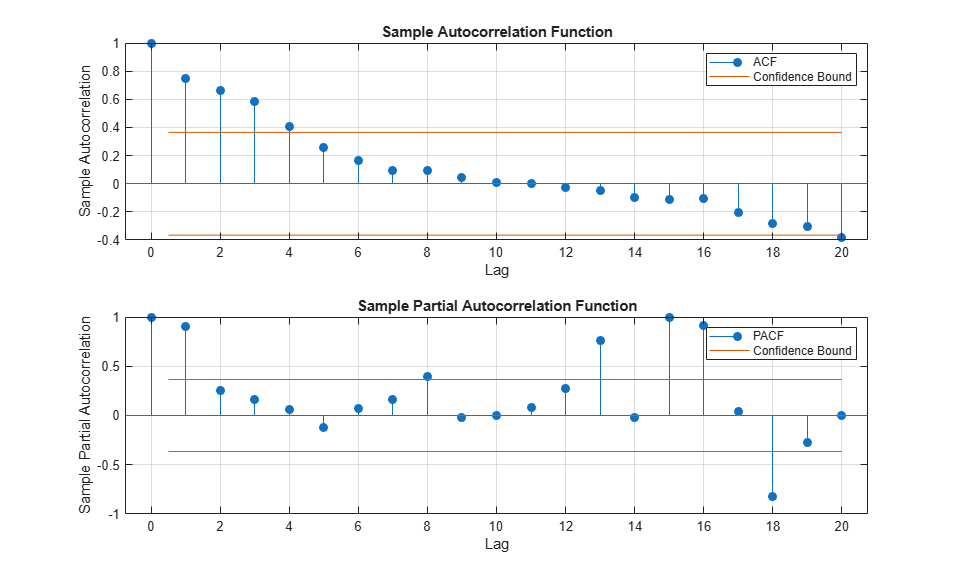## Assess Stationarity of a Time Series

This example shows how to check whether a linear time series is a unit root process in several ways. You can assess unit root nonstationarity statistically, visually, and algebraically.

### Simulate Data

Suppose that the true model for a linear time series is

``

where the innovation seriesis iid with mean 0 and variance 1.5. Simulate data from this model. This model is a unit root process because the lag polynomial of the right side has characteristic root 1.

```SimMod = arima('AR',0.2,'MA',-0.5,'D',1,'Constant',0,... 'Variance',1.5); T = 30; rng(5); Y = simulate(SimMod,T); ```

### Assess Stationarity Statistically

Econometrics Toolbox™ has four formal tests to choose from to check if a time series is nonstationary: `adftest`, `kpsstest`, `pptest`, and `vratiotest`. Use `adftest` to perform the Dickey-Fuller test on the data that you simulated in the previous steps.

```adftest(Y) ```
```ans = logical 0 ```

The test result indicates that you should not reject the null hypothesis that the series is a unit root process.

### Assess Stationarity Visually

Suppose you don't have the time series model, but you have the data. Inspect a plot of the data. Also, inspect the plots of the sample autocorrelation function (ACF) and sample partial autocorrelation function (PACF).

```plot(Y); title('Simulated Time Series') xlabel('t') ylabel('Y') subplot(2,1,1) autocorr(Y) subplot(2,1,2) parcorr(Y) ```The downward sloping of the plot indicates a unit root process. The lengths of the line segments on the ACF plot gradually decay, and continue this pattern for increasing lags. This behavior indicates a nonstationary series.

### Assess Stationarity Algebraically

Suppose you have the model in standard form:

``

Write the equation in lag operator notation and solve forto get

``

Use `LagOp` to convert the rational polynomial to a polynomial. Also, use `isStable` to inspect the characteristic roots of the denominator.

```num = LagOp([1 -0.5]); denom = LagOp([1 -1.2 0.2]); quot = mrdivide(num,denom); [r1,r2] = isStable(denom) ```
```Warning: Termination window not currently open and coefficients are not below tolerance. r1 = logical 0 r2 = 1.0000 0.2000 ```

This warning indicates that the resulting quotient has a degree larger than 1001, e.g., there might not be a terminating degree. This indicates instability. `r1 = 0` indicates that the denominator is unstable. `r2` is a vector of characteristics roots, one of the roots is 1. Therefore, this is a unit root process.

`isStable` is a numerical routine that calculates the characteristic values of a polynomial. If you use `quot` as an argument to `isStable`, then the output might indicate that the polynomial is stable (i.e., all characteristic values are slightly less than 1). You might need to adjust the tolerance options of `isStable` to get more accurate results.